# Three Calculator Simulation Activities

beginning of content:

Here are three short simulation activities that teach fairly narrow topics in the AP Statistics curriculum. They are:

• A demonstration that if X and Y are independent, then Var(X + Y) = Var(X - Y) = VarX + VarY
• A demonstration of the normal probability plot
• A demonstration of what the t distribution represents and why we need it

Each of these, along with appropriate classroom discussion, is meant to take 20 minutes or less. While some simulations are best done incorporating tangible objects, such as tokens drawn from a bag, these simulations are designed for the graphing calculator alone. As an activity progresses, teachers should make sure that the students understand what the calculator is doing before guiding the discussion toward more abstract statistical principles.

The syntax throughout this article is that of the TI-83 or TI-84, the calculator models that are probably the most widely used in statistics classrooms. Of course, the activities may be done with any calculator or computer having basic random-number-generating functions. On the TI-83 and TI-84 calculators, the random-number-generating functions are located under the math → prb menu. The notation X ~ N(µ,σ) used in this document indicates that X is a random variable having a normal distribution with mean µ and standard deviation σ.

This is a short simulation demo I like to do with students to show (not prove) that variances add even when the random variables are subtracted, but only if they're independent.

Let X = the time it takes a lab rat to run one maze and Y = the time it takes the same rat to run a different maze. X and Y take different values for different rats and therefore have distributions over the population of rats. Let's suppose that X~N(13,4) and Y~N(10,3)—i.e., both variables have normal distributions, one with mean time 13 seconds and standard deviation 4 seconds, the other with mean time 10 seconds and standard deviation 3 seconds.

Let's further suppose that X and Y are independent of one another. Then, using the standard TI functions, we can simulate 100 different rats' maze runs X and Y with these commands:

randNorm(13,4,100)→L1
randNorm(10,3,100)→L2

It is instructive to make a scatterplot of (X,Y) before continuing. The scatterplot should show that X and Y are uncorrelated, as you would expect for independent random variables.

Next, calculate the mean and standard deviation of X and of Y. When I did this using 1-Var-Stats, I got the following: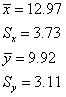Notice that the results don't match the input parameters exactly. Such discrepancies are expected when simulating with only 100 trials. If you did the calculations with more simulated values, you would expect the sample statistics to match the parameters more closely.

Okay, now let's put X + Y (the total time it takes a rat to run both mazes) and X - Y (how much longer a rat takes to run the first maze than the second) in lists L3 and LΑ:

L1+L2→L3
L1-L2→L4

Make histograms of L3 and L4. With the parameters I've proposed, you should be able to put them on the same graph, and they will overlap only a little. My window parameters are -20 < x < 50 , Xscl=2 and -10 < y < 40, Yscl=10.

Pay attention to where the means are first. You want them to be around 13 + 10 = 23 for X + Y and around 13 - 10 = 3 for X - Y. Now pay attention to the spreads. They should look about the same for the two graphs. We're just as uncertain about X + Y as about X - Y. Now let's calculate the mean and standard deviations of X + Y and X - Y for our simulated values. For my simulation, I obtained the following:

mean(L3)=22.89
stdDev(L3)=5.20
mean(L4)=3.05
stdDev(L4)=4.49

Using the formulas for adding variances of independent random variables, we would expect both of these standard deviations to be about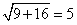, and they are. If we use the sample standard deviations for X and Y, we get a slightly different prediction. For my simulations, it's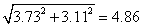, which is also close. If you do this with your students, some of them will get numbers closer to the prediction than others. If you check the sample means in the same way (i.e., compare the means of L3 and L4 with the sum and difference of the means of L1 and L2, respectively), you'll find that the match is always exact. That's because means for random variables always add exactly, regardless of the correlation. The reason our variances don't quite add up like we think they ought to is that in our simulations, we didn't get X and Y that were perfectly uncorrelated. Indeed, if you have students for whom the standard deviations of L3 and L4 are quite a bit different from 5, chances are that they happened to get a simulated set of Xs and Ys that had more correlation to them than did the other students'. A scatterplot of L1 and L2 might show this.

Now let's suppose that in fact the rats' times are positively correlated. That is, if a rat runs one maze faster than most rats, then it's likely to run the other maze faster than most rats as well. One way to simulate this (it's somewhat crude, but it will suffice for this demo) is to keep the same simulated values we just had, but now sort both L1 and L2 in ascending order so that the rat that ran the first maze fastest of all is also the rat that ran the second maze fastest of all, and so on. Do this, and also recompute L3 and L4 as the sum and difference of L1 and L2 respectively.

sortA(L1)
sortA(l2)
L1+L2→L3
L1-L2→L4

A scatterplot of L1 and L2 will now, of course, show a very strong correlation. The means and standard deviations of these lists will be the same as they were before, since the numbers in the list are the same. But what about the means and standard deviations of L3 and L4? Make a histogram of them as before, and you should see that the sum X + Y has a lot more variability in it than the difference X - Y. The reason is, since rats that run one maze faster also tend to run the other maze faster, then subtraction tends to subtract out the "rat effect," leaving behind only the "maze difficulty effect." (Incidentally, this is a good time to plant the seed of understanding for paired t-tests with your students.) But adding X and Y does not subtract out the "rat effect." It just lumps one uncertainty with the other, creating more uncertainty.

If you compute the means and standard deviations for L3 and L4, you should find that the means have remained unchanged from before—the fact that E(X + Y)=E(X)+E(Y) and E(X - Y)=E(X) - E(Y) doesn't require independence of X and Y. But the standard deviation (and hence the variance) of X + Ywill be larger than before—the standard deviation will be larger than 5—and the standard deviation (and hence the variance) of X - Y will be smaller than before—the standard deviation will be less than 5. On my calculator, I got stdDev(L3)=6.82 and stdDev(L4)=0.86. Lesson: When X and Y are correlated, the variances don't add for either X + Y or X - Y.

Okay, here's a final, optional piece of this demo that you can include if you like, though it isn't necessary for getting across the main idea. (For some students, it may even cloud the main issues and should be excluded.) What if X and Y are negatively correlated? (This is probably unrealistic and is included to demonstrate a statistical, not a biological, principle. But perhaps a plausible mechanism for negative correlation is that rats may tend to be either "Quick Starters" or "Timids." The Quick Starters will tire and run the second maze slower than they did the first, and the Timids will acquire more confidence with the second maze and run it faster than they did the first.) Let's simulate a negative correlation by keeping the values in L1 as they are but sorting L2 in descending order:

sortD(L2)
L1+L2→L3
L1-L2→L4

A scatterplot of L1 and L2 shows the negative correlation. Histograms of L3 and L4 now show the opposite pattern from before: X + Y has very little variability compared with X - Y. It's no wonder: with the Quick Starters slowing down on the second maze and the Timids speeding up, all the rats now tend to take about the same total time to run the two mazes. But the differences are now seriously affected, because a rat who ran the first maze slowly will tend to run the second maze faster, exaggerating this further in the difference X - Y. Checking stdDev(L3) and stdDev(L4) confirms this.

## Activity 2: Normal Probability Plots

This activity is meant to help students understand what they should look for when using normal probability plots to assess normality. The details of how to construct a normal probability are somewhat complicated (and not universally agreed upon), but happily, AP Statistics students need not concern themselves with these details.

### A. Large Normal Data Sets

Let's first look at a large set of simulations drawn from a normal distribution. The following command will store into list L1 a set of 100 simulated draws of men's heights, taking the distribution to be normal, with a mean of 70 inches and a standard deviation of 3 inches. Have your students all do this on their calculators.

randNorm(70,3,100)→L1

Now ask every student to make a histogram of his or her values. A reasonable window is 60 < x < 80, Xscl=2,0 < y < 30,Yscl=10. (My students want to use the "zoom stat" feature, but that is not a good idea for histograms.) Some histograms will look rather normal, while others will not. Look at some of the histograms they made, and make a note of any students whose graphs show an upper-end or lower-end outlier. Ask the students to share their plots with their neighbors.

Now ask your students to make a normal probability plot of their values. That is the lower-right plotting option icon on the TI-83 and TI-84 calculators. The default location for the data axis is X, but I prefer Y, as it allows easier interpretation of deviations from normality, as will be discussed shortly.

Most of the students will have plots that look quite linear. That's as it should be, and one of the points of this lesson is that linearity in a normal probability plot indicates approximate normality in the data. A few may have "blips" at one or both of the tails of their plots, particularly upper-end values that seem too high and lower-end values that seem too low. These correspond to the outliers we saw in our histograms. But it is important for the students to recall that the values really were simulations from a normal distribution, so these outliers are actually to be expected. They usually are not so grossly far out that they suggest a heavy-tailed or skewed distribution.

### B. Large Nonnormal Data Sets

It's a good idea for students to see what normal probability plots look like for data that do not come from a normal distribution. You can simulate 100 values from an exponential distribution, which is right-skewed, with the following command:

-ln(rand(100))→L1

(I am not including here the details of why this simulates values from an exponential distribution, but a brief explanation may be in order. "rand(100)" generates 100 simulated random variables drawn from the distribution that is uniform over the interval [0,1]. Taking the natural logarithm of those values transforms them to the interval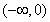while also "stretching out" the distribution, creating the skew desired for this demonstration. And negating them, of course, transforms them to the interval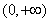, which is the domain of the skewed exponential distribution.)

Have your students do this and make histograms and then normal probability plots. They should see very clear right skew in the histograms. In the normal probability plots, they should see very clear curvature, indicating nonnormality. In particular, they should see a plot that is concave up, indicating right skew. I mentioned earlier that I like to put the data on the Y-axis. My reason for this is that one can think of a diagonal line through the normal probability plot as being a "prediction" of what the data should look like if the distribution is normal. In the plot for these right-skewed data, the upper values would be above the line, indicating that the high values were "too high" to be normal. When high values are "too high," that indicates right skew. You can simulate left-skewed numbers with the following command, and its normal probability plot should reveal the left skew in that the low numbers will be "too low":

2+ln(rand(100))→L1

### C. Small Normal Data Sets

It's important for students to understand that when they are performing inference and want to check the assumption that their data come from a normal distribution, there is no tool that will positively verify the assumption. The assumption may be demonstrated false with a normal probability plot, or perhaps a histogram or a boxplot, but there is no way to verify that it is true. All these diagnostic tools can do is verify that the assumption of normality is reasonable. These checks should be made, of course, but the assumption of an approximately normally distributed population remains an assumption.

With small data sets, a normal probability plot may look rather nonlinear, even if the data really do come from a normal distribution. In this last part of this activity, we simulate small data sets from a normal distribution and see what sorts of normal probability plots are typical. The following command will store into list L1 the simulated heights in inches of 10 men:

randNorm(70,3,10)→L1

Have your students do this and make a normal probability plot of their results. Have them share their plots with their neighbors. Then have them repeat the simulation several more times, looking each time at the normal probability plot they get. They should see that although roughly linear plots are common (the data were simulated from a normal distribution, after all), it is not that unusual to see some rather large deviations from linearity in the normal probability plot. The moral is that with small data sets, a certain amount of nonlinearity is to be expected even for data that really do come from a normal distribution; that is the nature of sampling variability. Unless these deviations are gross, they do not necessarily invalidate the assumption of normality that is required for some inference techniques.

## Activity 3: Introducing the t Distribution

This activity introduces students to the t distribution family. It is in several steps.

First, we simulate the height of an adult American male, assuming the population to be normal with mean 70 inches and standard deviation 2.6 inches, which isn't too far from truth.

randNorm(70,2.6)

Then we simulate three at a time:

randNorm(70,2.6,3)

You have to scroll to the right after doing this in order to see all three heights in the list. Now it gets a little bit tricky. On the TI-83 and TI-84, the colon (same button as the decimal) can be used to separate commands that are entered on a single line. The output you see is the result of the last command. (For example, 1→X:X+1 would report back "2".) So use the following command to (1) simulate three men's heights, and then (2) compute the standardized z-score for the sample mean, given that the population mean is 70 and the population standard deviation is 2.6. The function mean( ) on the TI-83 is located under the 2nd-list-math menu.

randNorm(70,2.6,3)—>L1:(mean(L1)-70)/(2.6/sqrt(3))

The reason the commands are separated by a colon rather than entered separately is to allow students to repeat the simulation quickly and easily simply by pressing the Enter button repeatedly. The Enter button, when pressed after no new commands are entered, reexecutes the last instruction line.

Have your students press Enter a few times to get a feel for the sort of numbers it produces. Despite having studied the topic, many students do not immediately see that the numbers produced by this simulation should have a standard normal distribution. It helps to write on the board the same computation in correct notation, with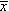,µ, and ,var>σ, and then substitute in 70 and 2.6: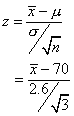Now ask your students to press Enter repeatedly, which will repeat the entire simulation many times. Any time they see a number that is larger in magnitude than 3, they are to say it out loud. You are likely to hear an occasional "3.1" or "-3.3", but numbers much farther from zero will be quite rare. No students are likely to see any numbers greater in magnitude than 4.

The last step is to replace 2.6 in the expression above with stdDev(L1), a quantity that depends upon the "data." This is equivalent to replacing σ of the population with its estimate s, computed from a sample. The function stdDev( ) on the TI-83 is located under the 2nd-list-math menu.

randNorm(70,2.6,3)→L1:(mean(L1)-70)/( stdDev(L1)/sqrt(3))

Once again, ask your students to say out loud any numbers they see that are greater in magnitude than 3. You will almost certainly hear several 4s, a 6, perhaps even a 12—numbers that would be unheard of from a standard normal distribution. (Numbers greater than 12 are rare even in a t distribution with 2 degrees of freedom, but not with 20 students simulating this 50 or so times each.)

Now do this yourself on the overhead calculator several times until you get a pretty large number, say a 7 or larger, and then stop. Ask your students what they expect to see in list L1. What makes the value of the standardized mean so big? There are two possible explanations: the sample mean is pretty far from the true mean of 70, or else the sample standard deviation is pretty small (or, more likely, both). After they've thought about it and perhaps given those answers, look in list L1. Very likely, you will find three numbers that are all at least an inch away from 70 inches, and all in the same direction, and they will likely be fairly close to one another, making s relatively small.

The point of this is to convince students that the distribution of the t statistic is more spread out than the normal distribution, and the reason is that you're dividing by a random quantity s that may vary a lot when the sample size is small. The variability in s is what creates the heavy tails in the t distribution. The reason the distribution begins to look more normal when the sample size gets larger is that the variability in s decreases.

If you repeat this activity with larger and larger sample sizes, you should have fewer and fewer students saying large numbers out loud.

Floyd Bullard received his bachelor's degree in mathematical sciences from the Johns Hopkins University in 1991 and his master's degree in statistics from the University of North Carolina at Chapel Hill in 1999. He has taught high school math as a Peace Corps volunteer in Benin, West Africa, at the Horace Mann School in New York, and most recently at the North Carolina School of Science and Mathematics (NCSSM) in Durham, North Carolina. He is now on a leave of absence from NCSSM to study in a doctoral program in statistics at Duke University, after which he plans to return to teaching. Floyd is a Reader for the AP Statistics Exam.

## Authored by

• Floyd Bullard
North Carolina School of Science and Mathematics
Durham, North Carolina